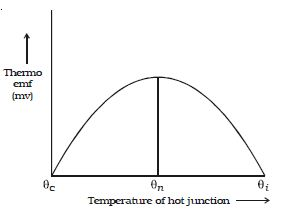# Explain on Neutral and Inversion Temperature

Neutral temperature is the temperature of the hot junction of a thermocouple at which the electromotive force of the thermocouple attains its utmost value when the cold junction is maintained at a stable temperature of 0°C. The graph showing the variation of thermo emf with the temperature of the hot junction, taking the temperature of the cold junction (θC) as the origin is shown in Figure. For a small difference in temperature between the junctions, the graph is a straight line. For a large difference in temperature, the graph is a parabola.Fig: Graph showing the variation of thermo emf with temperature

Keeping the temperature of the cold junction constant, the temperature of the hot junction is gradually increased. The Thermo emf rises to a maximum at a temperature (θn) called neutral temperature and then gradually decreases and eventually becomes zero at a particular temperature (θ1) called inversion temperature. Beyond the temperature of inversion, the Thermo emf changes sign and then increases.

For a given thermocouple, the neutral temperature is a constant, but the temperature of inversion depends upon the temperature of the cold junction. These temperatures are related by the expression

C – θ1)/2 = θn